## October 09, 2003

### Mean-Variance Analysis

Comparing securities using means and variances.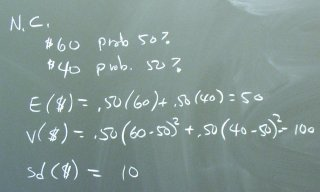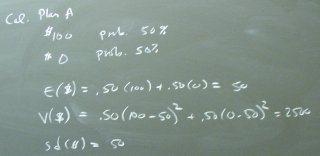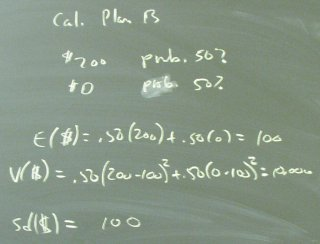The general rules: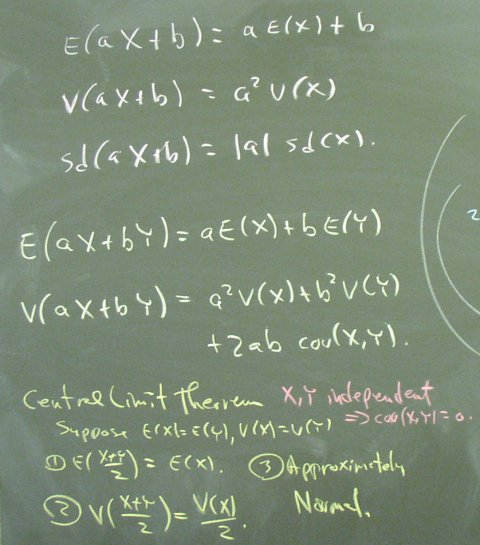A demonstration of why the rules are true: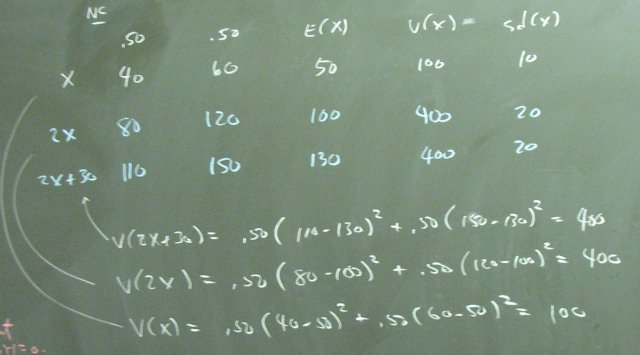Rule: V(X+Y) = V(X) + V(Y) + 2 COV(X,Y).

Proof: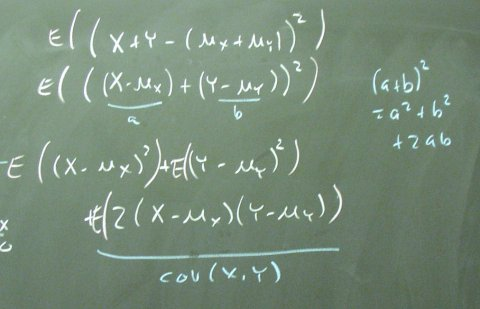Pictures of covariances and correlations: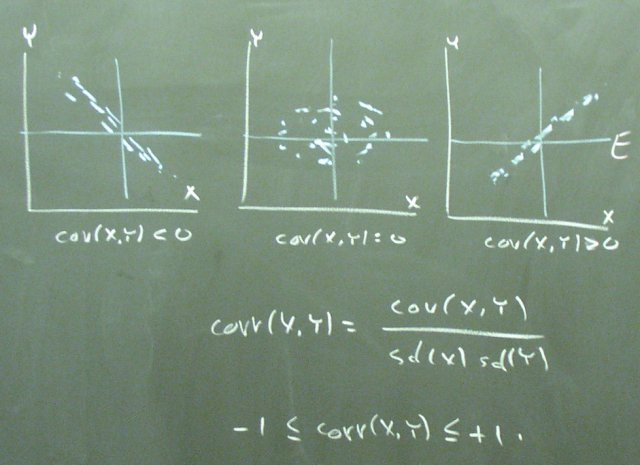Question: Can investors exploit this last rule and/or the Central Limit Theorem to get favorable combination of risk and return?

Posted by bparke at 04:36 PM